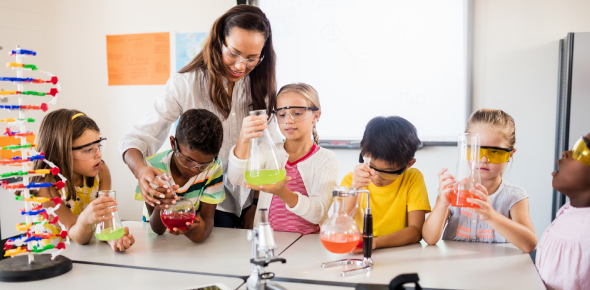# Ks3 Science Quiz Questions And Answers

30 Questions | Attempts: 21001SettingsWelcome to the ultimate KS3 (Key Stage 3) Science quiz. It's a perfect practice test for students in Year 7, Year 8, and Year 9. These 30 questions, cover the fundamental topics of science. So, if you are interested in having a practice session, then you need to take this quiz right now! Learn new things that you might not have known or just revise what you already know, this quiz is perfect for both these purposes!

• 1.
What is the word equation for respiration ?
• A.

Carbon Dioxide + water = Glucose + Oxygen

• B.

Water + Oxygen = Hydrogen peroxide + hydrogen

• C.

Oxgen + Glucose = Water + Carbon dioxide + Energy

• 2.
What is the role of the liver in the human body?
• A.

To break down toxins and store food

• B.

To break down blood

• C.

To defend the body from radiation

• 3.
What type of force prevents a football from rolling on the grass forever?
• A.

Gravity

• B.

Uphtrust

• C.

Friction

• D.

Neutrons

• 4.
When light travels through materials of different densities this may be observed?
• A.

Reflection

• B.

Rebound

• C.

Refraction

• D.

Line of Incidence

• 5.
What fluid protects a baby during development?
• A.

Amniotic fluid

• B.

Watery fluid

• C.

Grey fluid

• 6.
What type of energy is stored in a battey?
• A.

Kinetic energy

• B.

Gravitational potential energy

• C.

Light energy

• D.

Chemical energy

• 7.
If an incident light ray produces an angle of incidence equal to 30 degrees when hitting a mirror: What is the angle of reflection equal to?
• A.

90 degress

• B.

30 degrees

• C.

180 degress

• D.

45 degress

• 8.
These sorts of rocks are created due to extreme heat and pressure.
• A.

Metamorphic rock

• B.

Igneous rock

• C.

Sedimentary rock

• 9.
What do red blood cells transport in the human body?
• A.

Oxygen and nutrients

• B.

Carbon dioxide and battery acid

• C.

Stem cells are and antibodies

• 10.
Respiration is performed by both animals and plants to make energy: What are the waste products created by a human during respiration?
• A.

Water and glucose

• B.

Oxygen and water

• C.

Carbon Dioxide and Water

• 11.
What is Mass?
• A.

How heavy something is and measured in grams or kilograms

• B.

The force of gravity on an object emasured in newtons

• C.

The density of an object

• D.

The volume of an object

• 12.
A shirt can be seen as the color red because?
• A.

All the colors of the color spectrum are absorbed

• B.

The color red is reflected to the eye and other colors are absorbed

• C.

The colour red is absorbed by the shirt

• 13.
When a substance reaches the freezing point a liquid substance will start to turn into...
• A.

Gas

• B.

Solid

• C.

Liquid

• D.

Dark matter

• 14.
When a substance reaches the boiling point what physical change usually takes place?
• A.

Liquid to gas

• B.

Gas to liquid

• C.

Solid to gas

• 15.
The temperature of water at the freezing point and the melting point is?
• A.

100 degrees

• B.

0 degrees

• C.

50 degrees

• 16.
The following acids listed should produce these salts when reacted with magnesium metal. Hydrochloric acid Nitric acid Sulphuric acid
• A.

Hydrogen Sulphate, Magnesium Sulphate, Magnesium Chloride

• B.

Magnesium Chloride, Magnesium Nitrate, Magnesium Sulphate

• C.

Magnesium solution, magnesium acid, Magnesium metal

• 17.
Which of these major nutrients provides the most energy per gram?
• A.

Protein

• B.

Carbohydrate

• C.

Fat

• 18.
Which of these nutrients is needed for growth and repair of body tissue?
• A.

Carbohydrate

• B.

Protein

• C.

Fats

• 19.
Salt and water mixed together is an example of...
• A.

A Compound

• B.

An Element

• C.

A Mixture

• 20.
Magnesium, Oxygen, Carbon, Neon and Hydrogen are all single words and represent?
• A.

Compounds

• B.

Element

• C.

Mixtures

• 21.
When Iron and Sulphur are mixed together and heated a new substance is formed called Iron Sulphide this is an example of?
• A.

A compound

• B.

A element

• C.

A mixture

• 22.
The amplitude measures?
• A.

The oscillations in a wave as pitch

• B.

The height of a wave as sound

• C.

The speed of a wave as wavelength

• 23.
The frequency measures?
• A.

The number of oscillations in a wave as spitch

• B.

The number of peaks in a wave as wavelength

• C.

The speed of a wave as frequency

• 24.
Which color in the color spectrum has the longest wave-length?
• A.

Red

• B.

Yellow

• C.

Blue

• 25.
Which color in the color spectrum has the shortest wave-length?
• A.

Yellow

• B.

Violet

• C.

Red

## Related TopicsBack to top
×

Wait!
Here's an interesting quiz for you.